TitleCollege Algebra
Tutorial 33: Practice Test on Tutorials 25 - 32Learning Objectives

 After completing this tutorial, you should be able to: Take a test on topics covered in tutorials 25 - 32 in this website.

 Special Notes about Tutorial 33: I can not guarantee you will pass your test after going though any of the tutorials in this website or this practice test. However, it will definitely help you to better understand the topics covered better. Disclaimer: WTAMU and Kim Seward are not responsible for how a student does on any test or any class for any reason including not being able to access the website due to any technology problems.  There are no videos on this page. To view videos on the type of problems found on this page, please go to the tutorial (25 - 32) that corresponds with that problem.Introduction

It is important to note that, chances, are I'm not your math instructor.  You need to check with your math teacher as to things like when your next math test is and what it covers.  It may cover more material on the test than what is in this practice test.  Just note that there are other practice tests at this website. So, after finding out what is on your test (if you have one) do the practice test(s) problems that go with the test you are preparing for.  If you are not in a class or are not having a test soon, this practice test is still good practice to go through and check to make sure you are understanding this material before moving on - kind of like a spot check.  The material on this practice test goes with Tutorial 25:  Slope of a LineTutorial 26:  Equations of Lines, Tutorial 27:  Graphing Lines, Tutorial 28:  Parallel and Perpendicular Lines, Tutorial 29:  Circles, Tutorial 30:  Introduction to Functions, Tutorial 31:  Graphs of Functions, Part I, Tutorial 32:  Graphs of Functions, Part II.

Also note that your teacher may word the problems on their test a little differently, may have some different kinds of problems, or may have a different number of problems than what is in this practice test.  Again, since I'm probably not your math instructor, I don't know exactly how your teacher will set up your math test.  Just note that these problems will help you build an understanding of the concepts presented and the terms used in math problems.  If you have an understanding of the problems instead of just memorizing them, then you should do fine on these concepts, no matter how the test is set up.

 Steps to Studying for a Math Test
1. Work through problems.  If you are in a class, you should have done this on completion of any homework you have done.  For anyone,  you can accomplish this by doing the practice problems found in each tutorial.
2. Check work on problems.  The practice problems in each tutorial have links to the answers to them so you can instantly check how you are doing.  Also, in most math books, the odd answers are found in the back of the book.
3. Review concepts.  Whether you got the problems right or wrong, make sure you review over them.  If you did get a problem wrong, make sure you either review that concept in it's respective tutorial or ask your math teacher about it.  If you don't ask about a problem before a test,  you are going to kick yourself when it comes up on the test.
4. Work through problems as if you were taking the test - no notes, book, webpages, etc.  This practice test is a perfect way to do that. After taking this practice test, check your answers by clicking on the link to the answer key found at the bottom of the practice test (before the 'need extra help on these topics' section)

 During the Test

It is to your benefit to show as much of the work as possible on the problems that have several steps involved.

Make sure that you read the directions carefully,  you wouldn't believe how many points get taken off  math tests for people not following directions.

Pace yourself.  You do not have to be the first one done to do well on the test.  Do not panic if there is still time left to take the test and others are turing it in.  Sometimes that means they do not know the material and left some of the answers blank.  Do not worry about anyone else but yourself.

Don't rush through a problem.  Another thing that math teachers take points off for are careless mistakes made by people that rush through a problem.  When those students get their tests back, they bonk themselves on the head at some of the things that got counted wrong,  things that they knew how to do.

Remember to breathe!!!!  I know some of you are scared to death at the thought of having to take a math test of any kind.  For you guys, try to relax and don't forget to breathe.  (Even if you aren't scared to take a math test,  it is probably a good idea to remember to breathe,  I wouldn't want you to pass out during the test). If it feels like your brain has left the building during your test, just close your eyes and breathe in and out and in and out and your brain will return.

Good luck on your test.  If you are taking a math test soon, don't panic, you are going to do great!!!Practice Test

 Problems 1a - 1c:  Find the slope of the straight line that passes through the given points or state that the slope is undefined.  Then indicate if the line through the points rises (left to right), falls (left to right), is horizontal, or is vertical.

 1a.  (7, 4) and (1, 1) 1b.  (-3, 4) and (2, -1)

 1c.  (-5, 3) and (-5, 2)

 Problems 2a - 2d:  Write an equation for the line in point/slope form and slope/intercept form that has the given condition.

 2a.  Slope = 3/2 and passes through the origin.

 2b.   x-intercept = 4 and y-intercept = -3

 2c.  Passes through (3, 2) and is parallel to.

 2d.  Passes through (-1, -1) and is perpendicular to.

 Problems 3a - 3d:  Give the slope and y-intercept of the given line and then graph it.

 3a.3b.3c.3d.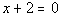Problems 4a - 4b:  Find the slope of the line that is a) parallel and b) perpendicular to the given line.

 4a.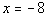4b.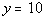Problem 5a: Write the standard form of the equation of the circle with the given center and radius.

 5a.  center (-2, 0) and r = 3

 Problem 6a:  Find the center and radius of the given circle and graph it.

 6a.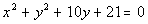Problems 7a - 7b:  Determine if the given relation is function or not.  Give its domain and range.

 7a.  {(-1, 2), (1, 3), (-1, 4), (2, 5)} 7b.  {(1, 1), (2, 2), (3, 3), (4, 4)}

 Problems 8a - 8b:  Decide whether y is a function of x.

 8a.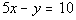8b.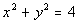Problem 9a:  Find and simplify a)  f(a),  b)  f(a + h) and  c)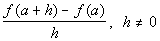using the given function.

 9a.Problem 10a:  Find the functional values f(-5), f(1), and f(3) for the compound function.

 10a.Problems 11a - 11c:  Give the domain of the function.

 11a.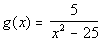11b.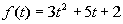11c.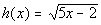Problems 12a - 12e:  Graph the given function using the given values of x.   Also use the graph to determine the domain and range of  the function.

 12a.;   x = -3, -2, -1, 0, 1, 2, 3

 12b.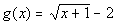; x = -1, 0, 3, 8

 12c.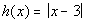;  x = 0, 1, 2, 3, 4, 5, 6

 12d.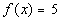; x = -3, -2, -1, 0, 1, 2, 3

 12e.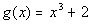;  x = -2, -1, 0, 1, 2

 Problem 13a:  Use the graph to determine a) the x-intercepts, if any b) the y-intercept, if any, c) the functional value indicated, to determine intervals on which the function is d) increasing, if any, e) decreasing, if any, and f) constant, if any.

 13a.Problems 14a - 14b:  Use the vertical line test to identify graphs in which y is a function of x.

 14a.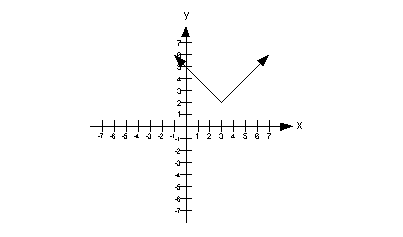14b.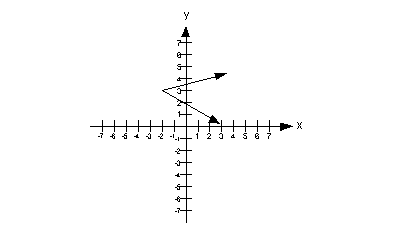Problems 15a - 15b:  Determine if the given function is even, odd or neither.

 15a.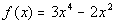15b.Problem 16a: If f(x) = int(x), find the given functional value.

 16a.  f(-14.321)

 Now you are ready to check the answers to your practice test:Need Extra Help on these Topics?

The following are web pages that can assist you in the topics that were covered on this page:

Go to Get Help Outside the Classroom found in Tutorial 1: How to Succeed in a Math Class for some more suggestions.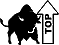Last revised on July 3, 2010 by Kim Seward.
All contents copyright (C) 2002 - 2010, WTAMU and Kim Seward. All rights reserved.